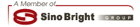| 加到我的最愛 | 網頁指南 | ENG |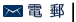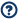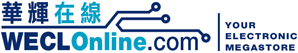首頁 關於華輝 最新推廣 門市優惠 牌子分類 資源共享 保用登記 聯絡我們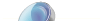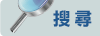By Keyword By Model Number By Brand By Product Name By Product Code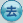產品目錄分類門禁／警報系統線材及配件公共天線／數碼廣播系統閉路電視／錄影系統接駁器及配件消費電子產品電器／電池及供電器材電子零件電腦及網絡配件廣播／對講系統測試器材及儀錶工具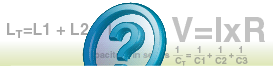Useful FormulaUseful Formulae: V = I x R P = V x I = V2/R Resistance: RT = R1 + R2 + R3 ……(Series) 1/RT = 1/R1 + 1/R2 + 1/R3 ……(Parallel) Capacitance: 1/CT = 1/C1 + 1/C2 + 1/C3 ……(Series) CT = C1 + C2 + C3 ……(Parallel) Q = it Inductance: LT = L1 + L2 + L3 …… (Series) 1/LT = 1/L1 + 1/L2 + 1/L3 ……(Parallel) Circuit With Capacitor & Resistor: E = iR + 1/C∫idt (Series) Circuit With Resistor & Inductor: E = iR + L x di/dt (Series)ResourcesAbbreviationUseful FormulaCalculatorMaster CatalogueFirmware DownloadRoHS CompliantSimulator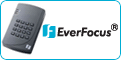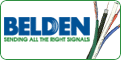【營業時間: 星期一至星期六 (10:30 – 19:00) ; 公眾假期 (12:00 – 19:00) ; 星期日 (休息)】

【辦公時間: 星期一至星期五 (9:00 – 18:30) ; 星期六、日及公眾假期 (休息)】